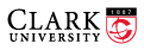Math 217/Econ 360, Probability and Statistics Fall 2014 Prof. D. Joyce, BP 322, 793-7421 Department of Mathematics and Computer Science Clark University

Pierre-Simon Laplace (1749–1827)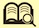Portrait of Laplace at the Palace of Versailles
Date 1842, posthumous portrait by Sophie Feytaud

`[This course page is obsolete.  I'll update it next time I teach the course.]`

Course information

• General description. An introduction to probability theory and mathematical statistics that emphasizes the probabilistic foundations required to understand probability models and statistical methods. Topics covered will include the probability axioms, basic combinatorics, discrete and continuous random variables, probability distributions, mathematical expectation, common families of probability distributions, and the central limit theorem.
This course is cross-listed as Econ 360.

• Prerequisites. Math 130 Linear Algebra, and Math 131 Multivariate Calculus
• Course goals.
• To provide students with a good understanding of the theory of probability, both discrete and continuous, including some combinatorics, a variety of useful distributions, expectation and variance, analysis of sample statistics, and central limit theorems, as described in the syllabus.
• To help students develop the ability to solve problems using probability.
• To introduce students to some of the basic methods of statistics and prepare them for further study in statistics.
• To develop abstract and critical reasoning by studying logical proofs and the axiomatic method as applied to basic probability.
• To make connections between probability and other branches of mathematics, and to see some of the history of probability.

• Syllabus
• Basic combinatorics. Additive and multiplicative principles, permutations, combinations, binomial coefficients and Pascal’s triangle, multinomial coefficients
• Kolmogorov’s axioms of probability. Events, outcomes, sample spaces, basic properties of probability. Finite uniform probabilities. Philosophies of probability.
• Conditional probability. Bayes’ formula, independent events, Markov chains
• Random variables. Discrete random variables/distributions, expectation, variance, Bernoulli and binomial distributions, geometric distribution, negative binomial distribution, expectation of a sum, cumulative distribution functions
• Continuous random variables. Their expectation and variance. Uniform continuous distributions, normal distributions, Poisson processes, exponential distributions; gamma, Weibull, Cauchy, and beta distributions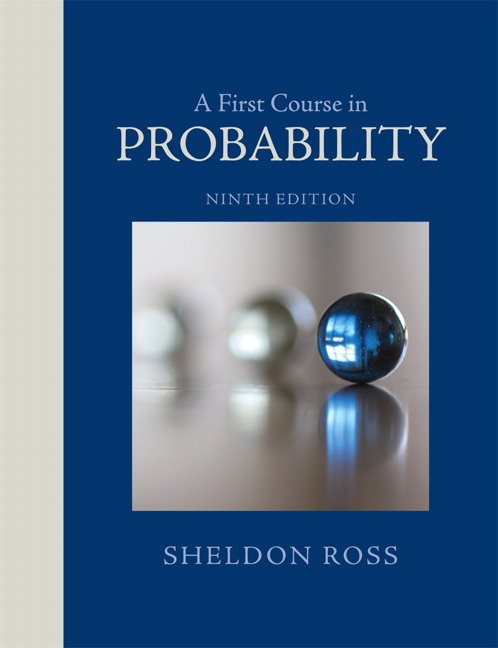• Joint random variables. Their distributions, independent random variables, and their sums. Conditional distributions both discrete and continuous, order statistics
• Expectation. Of sums, sample mean, of various distributions, moments, covariance and correlation, conditional expectation
• Limit theorems. Chebyshev’s inequality, law of large numbers, central limit theorem

• Textbook. A First Course in Probability, 9th edition, by Sheldon Ross. Pearson, 2014.
We may occasionally also refer to an on-line textbook, Charles M. Grinstead and J. Laurie Snell’s Introduction to Probability, published by the American Mathematical Society, 1997, on-line at http://www.dartmouth.edu/~chance/teaching_aids/books_articles/probability_book/book.html ..

• Course Hours. MWF 9:00–9:50.

• Assignments & tests. There will be numerous short homework assignments, mostly from the text, occasional quizzes, two tests during the semester, and a two-hour final exam during finals week.

• Time and study. Besides the time for classes, you’ll spend time on reading the text, doing the assignments, and studying of for quizzes and tests. That comes to about five to nine hours outside of class on average per week, the actual amount varying from week to week.

• Course grade. [tentative] The course grade will be determined as follows: 2/9 assignments and quizzes, 2/9 each of the two midterms, and 1/3 for the final exam.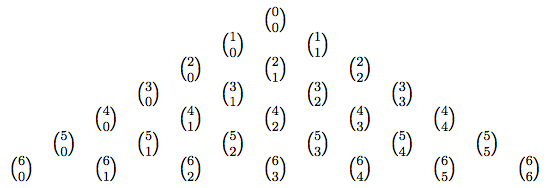Class notes, quizzes, tests, homework assignments

The dates for the discussion topics and the assignments are tentative. They will change as the course progresses.

1. Monday, Aug 25. Welcome and introduction to the class
Intro to probability via discrete uniform probabilities. Symmetry. Frequency.
Simulations and random walks

2. Wednesday, Aug 27.
Background on sets. Unions, intersections, complements, distributivity, DeMorgan's laws. Product, power sets. Countable and uncountable infinities.
Combinatorics. Principle of inclusion and exclusion, multiplicative principle, permutations, factorials and Sterling's approximation
Permutation generating applet
Assignment 1 due next Wednesday.

3. Friday, Aug 29. Binomial coefficients.
Combinations, Pascal's triangle, multinomial coefficients, stars & bars, combinatorial proofsThe BinomialPlot and GaltonBoard applets

Monday, Sep 1. Labor Day. No classes

4. Wednesday, Sep 3. Axioms for probability distributions.
Probability mass mass functions for discrete distributions, density functions for continuous distributions. Cumulative distribution functions. Sample spaces, axioms, and properties.
Assignment 1 due.
Assignment 2 due next Wednesday.

5. Friday, Sep 5. Uniform finite probabilities
Odds. Repeated trials. Sampling with replacement. The birthday problem.
The Birthday applet

6. Monday, Sep 8. Proofs of properties of probability distributions from the axioms.
Conditional probability. Xox.

7. Wednesday, Sep 10.
More on conditional probability: definition of conditional probability, the multiplication rule
Assignment 2 due.
Assignment 3 due next Wednesday.

8. Friday, Sep 12. Bayes' formula. Examples, tree diagrams

9. Monday, Sep 15.
Independent events. Definition, product spaces, independence of more than two events, joint random variables, random samples, i.i.d. random variables

10. Wednesday, Sep 17.
The Bernoulli process. Sampling with replacement. Binomial distribution, geometric distribution, negative binomial distribution, hypergeometric distribution. Sampling without replacement
Assignment 3 due.
Assignment 4 due next Wednesday.

11. Friday, Sep 19.
Discrete random variables. Probability mass functions, cumulative distribution functions. Various graphs and charts
Expectation for discrete random variables. Definition, expectation for the binomial and geometric distributions. St. Petersburg paradox.

12. Monday, Sep 22.
Select the date for the first test. It will cover chapter 1 through most of chapter 4.
More on expectation. Properties of expectation.
Variance for discrete random variables. Definition and properties.

13. Wednesday, Sep 24.
Variance of the binomial and geometric distributions.
Assignment 4 due.
Assignment 5 due next Wednesday.

14. Friday, Sep 26.
Continuous probability. Monte Carlo estimates. Introduction to the Poisson process and the normal distribution. Statement of the central limit theorem.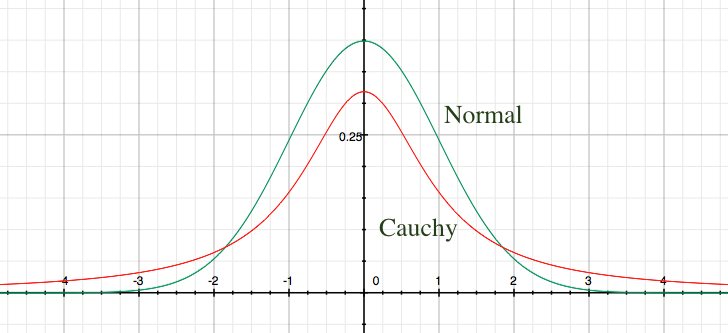15. Monday, Sep 29.
Density functions. Density as the derivative of the c.d.f., and the c.d.f. as the integral of density.

16. Wednesday, Oct 1.
Assignment 5 due.

17. Friday, Oct 3.
Examples of continuous distributions, functions of random variables, the Cauchy distribution.

18. Monday, Oct 6. Review for first test
Sample first test

19. Wednesday, Oct 8.

20. Friday, Oct 10.
The Poisson process. The Poisson, exponential, gamma, and beta distributions. Axioms for the Poisson process.

Monday, Oct 13. Fall break. No classes.

21. Wednesday, Oct 15.
Expectation and variance for continuous random variables. Definitions and properties. Expectation and variance for uniform and continuous distributions. Lack of expectation and variance for the Cauchy distribution.
Assignment 6 due next Wednesday.

22. Friday, Oct 17. The normal distribution, table for the c.d.f. of the standard normal distribution, the normal approximation to the binomial distribution. DeMoivre's 1733 proof for the first instance of the Central Limit Theorem.

23. Monday, Oct 20.
Joint distributions. Independent random variables. Joint c.d.f.'s and joint density functions.

24. Wednesday, Oct 22. Partial derivatives and multiple integrals relating to joint distributions.
Assignment 6 due.
25. Friday, Oct 24. Sums and convolution. The discrete case.
Assignment 7 due next Wednesday.

26. Monday, Oct 27. More on convolution. The continuous case. Gamma distributions as convolution of exponential distributions. Normal distributions convolute to other normal distributions.

27. Wednesday, Oct 29.
Conditional distributions. Conditional cumulative distribution functions, conditional probability mass functions, conditional probability density functions
Assignment 7 due.

28. Friday, Oct 31.
Covariance and correlation. Connection of covariance and variance, properties of covariance including bilinearity.
See Spurious Correlations to see that correlations often occur without associated causation.

29. Monday, Nov 3.
Order statistics

30. Wednesday, Nov 5. Review for second test
Sample second test

31. Friday, Nov 7. Second test. On 4.7, 5, and 6.1–6.5 (through conditional distributions). Answers. Extra answers.

32. Monday, Nov 10.
Moments and the moment generating function

33. Wednesday, Nov 12. Properties and examples of the moment generating function.

34. Friday, Nov 14. Joint probability distributions of multivariate functions, the Jacobian.
Notes from multivariate calculus about the Jacobians
Assignment 8 due next Wednesday.

35. Monday, Nov 17.
A proof of the central limit theorem

36. Wednesday, Nov 19. Discussion of statistical inference.

37. Friday, Nov 21.
Bayesian statistics Part I: an example, the basic principle, the Bernoulli process

38. Monday, Nov 24. Maximum likelihood estimators
Old notes from 218: Discrete case and Continuous case

39. Monday, Dec 1.
Bayesian statistics Part II: Bayes pool table, conjugate priors for the Bernoulli process, point estimators, interval estimators

40. Wednesday, Dec 3.
Bayesian statistics Part III: the Poisson process & its conjugate priors
Bayesian statistics Part IV: the normal distribution with known variance

41. Friday, Dec 5.
Bayesian statistics Part V: the normal distribution with unknown variance

42. Monday, Dec 8. Review.
Final will cover chapters 1–8 except sections 6.8, 7.6, 7.8, 7.9, 8.2, 8.4, 8.5. Also there will be an essay question on Bayesian statistics.
Sample final exam

43. Final exam.
Dec 16. 10:30. JC 220. Alternate Dec 12. morning, meet at my office sometime between 9 and noon.
Other information.

`http://aleph0.clarku.edu/~djoyce/ma217/`